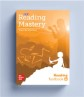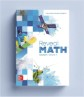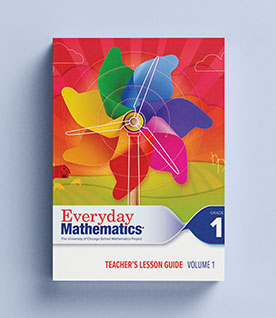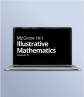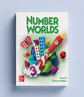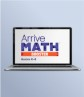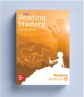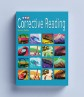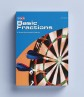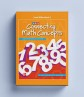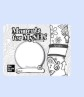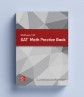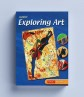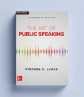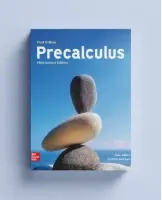## Our Principles# Distar Arithmetic 1992

Distar® Arithmetic carefully teaches basic math skills so students fully understand it and provides practice, ensuring student mastery. Students are shown how to use consistent, step-by-step thinking processes so all students can solve increasingly complex problems independently and with confidence.

## Program Details

The concept underlying Distar® Arithmetic is that virtually all children can learn if we teach them carefully. The basic math skills tasks are structured as simply as possible so that students fully understand each step.

Lessons emphasize thinking and understanding before memorization. Students are shown how to use consistent, step-by-step thinking processes so all students, even hard-to-teach children, can solve increasingly complex story problems independently and with confidence. That success can be very rewarding for them, and for you!

In Distar® Arithmetic I students will learn to:

• Work simple story problems that involve the operations above
• Work simple problems based on greater than and less than signs
• Apply ordinal counting to simple picture problems

In Distar® Arithmetic II students will learn to:

• Solve column-addition problems involving carrying
• Multiply single digit numbers
• Work regular multiplication and algebra-multiplication problems
• Work a variety of fraction problems
• Solve negative-number problems

Features:

• Beginning math skills are broken into sub skills that are taught one by one
• Sequence of skills is controlled so the students master all of the skills they need for later, more complicated tasks
• A single strategy is applied to a wide range of problems to emphasize thinking and understanding before memorization
• Frequent in-program mastery tests evaluate student's performance on critical skills